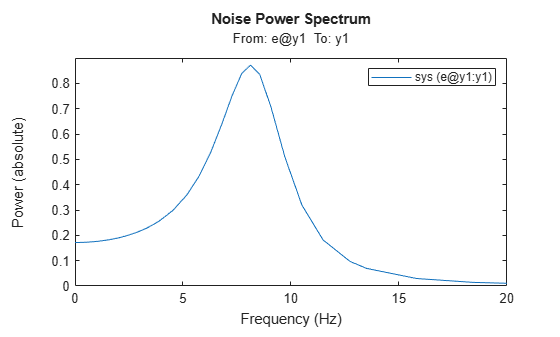spectrumplot

Plot disturbance spectrum of linear identified models

Syntax

spectrumplot(sys)
spectrumplot(sys,line_spec)
spectrumplot(sys1,line_spec1,...,sysN,line_specN)
spectrumplot(ax, ___)
spectrumplot(___,plot_options)
spectrumplot(sys,w)
h = spectrumplot(___)

Description

spectrumplot(sys) plots the disturbance spectrum of the model, sys. The software chooses the number of points on the plot and the plot frequency range.

If sys is a time-series model, its disturbance spectrum is the same as the model output spectrum. You generally use this function with time-series models.

spectrumplot(sys,line_spec) uses line_spec to specify the line type, marker symbol, and color.

spectrumplot(sys1,line_spec1,...,sysN,line_specN) plots the disturbance spectrum for one or more models on the same axes.

You can mix sys,line_spec pairs with sys models as in spectrumplot(sys1,sys2,line_spec2,sys3). spectrumplot automatically chooses colors and line styles in the order specified by the ColorOrder and LineStyleOrder properties of the current axes.

spectrumplot(ax, ___) plots into the axes with handle ax. All input arguments described for the previous syntaxes also apply here.

spectrumplot(___,plot_options) uses plot_options to specify options such as plot title, frequency units, etc. All input arguments described for the previous syntaxes also apply here.

spectrumplot(sys,w) uses w to specify the plot frequencies.

• If w is specified as a 2-element cell array, {wmin, wmax}, the plot spans the frequency range {wmin, wmax}.

• If w is specified as vector, the spectrum is plotted for the specified frequencies.

Specify w as radians/time_unit, where time_unit must equal sys.TimeUnit.

h = spectrumplot(___) returns the handle to the spectrum plot. You use the handle to customize the plot. All input arguments described for the previous syntaxes also apply here.

Input Arguments

 sys Identified linear model. line_spec Line style, marker, and color of both the line and marker, specified as a character vector. For example, 'b', 'b+:'. For more information, see Line Properties . ax Plot axes handle. Specify as a double-precision value. You can obtain the current axes handle by using the function, gca. plot_options Plot customization options. Specify as a plot options object. You use the command, spectrumoptions, to create plot_options. For more information, type help spectrumoptions. w Frequency range. Specify in radians/time_unit, where time_unit must equal sys.TimeUnit.

Output Arguments

 h Plot handle for spectrum plot, returned as a double-precision value.

Examples

collapse all

Obtain the identified model.

sys = ar(z9,4);

Plot the output spectrum for the model.

spectrumplot(sys);Obtain the identified model.

sys = ar(z9,4);

Specify the line width and marker style for the spectrum plot.

spectrumplot(sys,'k*--');'k*--', specifies a dashed line (--) that is black (k), with star markers (*).

Obtain multiple identified models.

sys1 = ar(z9,4);
sys2 = ar(z9,2);

Plot the output spectrum for both models.

spectrumplot(sys1,'b*-',sys2,'g^:');
legend('sys1','sys2');Obtain the axes handle for a plot.

sys1 = ar(z9,4);
spectrumplot(sys1);ax = gca;

ax is the handle for the spectrum plot axes.

Plot the output spectrum for another model on the specified axes.

sys2 = ar(z9,2);

hold on;
spectrumplot(ax,sys2,'r*--');

legend('sys1','sys2');Specify the plot options.

plot_options = spectrumoptions;
plot_options.FreqUnits = 'Hz';
plot_options.FreqScale = 'linear';
plot_options.Xlim = {[0 20]};
plot_options.MagUnits = 'abs';

Estimate an AR model.

sys = ar(z9,4);

Plot the output spectrum for the model.

spectrumplot(sys,plot_options);Obtain the identified model.

sys = ar(z9,4);

Specify the frequency range for the output spectrum plot for the model.

spectrumplot(sys,{1,1000});The 2-element cell array {1,1000} specifies the frequency range from 1 rad/s to 1000 rad/s.

Obtain the identified model.

sys = ar(z9,4);

Get the plot handle for the model spectrum plot.

h = spectrumplot(sys);(Optional) Specify the plot options, using the plot handle.

setoptions(h,'FreqUnits','Hz','FreqScale','linear','Xlim',{[0 20]},'MagUnits','abs');